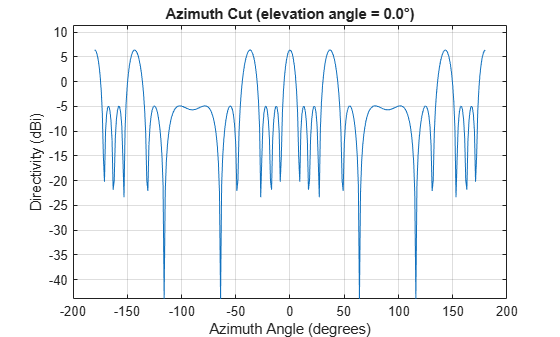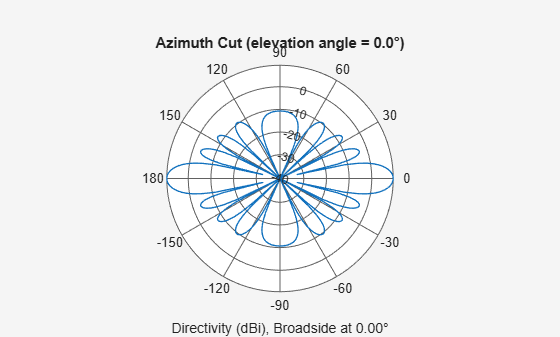Documentation

# step

System object: phased.ULA
Package: phased

Output responses of array elements

## Syntax

```RESP = step(H,FREQ,ANG) ```

## Description

### Note

Starting in R2016b, instead of using the `step` method to perform the operation defined by the System object™, you can call the object with arguments, as if it were a function. For example, `y = step(obj,x)` and `y = obj(x)` perform equivalent operations.

`RESP = step(H,FREQ,ANG)` returns the array element responses, `RESP`, at the operating frequencies specified in `FREQ` and in directions specified in `ANG`.

### Note

The object performs an initialization the first time the object is executed. This initialization locks nontunable properties (MATLAB) and input specifications, such as dimensions, complexity, and data type of the input data. If you change a nontunable property or an input specification, the System object issues an error. To change nontunable properties or inputs, you must first call the `release` method to unlock the object.

## Input Arguments

 `H` Array object `FREQ` Operating frequencies of array in hertz. `FREQ` is a row vector of length L. Typical values are within the range specified by a property of `H.Element`. That property is named `FrequencyRange` or `FrequencyVector`, depending on the type of element in the array. The element has zero response at frequencies outside that range. `ANG` Directions in degrees. `ANG` is either a 2-by-M matrix or a row vector of length M. If `ANG` is a 2-by-M matrix, each column of the matrix specifies the direction in the form ```[azimuth; elevation]```. The azimuth angle must lie between –180° and 180°, inclusive. The elevation angle must lie between –90° and 90°, inclusive. If `ANG` is a row vector of length M, each element specifies the azimuth angle of the direction. In this case, the corresponding elevation angle is assumed to be 0°.

## Output Arguments

 `RESP` Voltage responses of the phased array. The output depends on whether the array supports polarization or not. If the array is not capable of supporting polarization, the voltage response, `RESP`, has the dimensions N-by-M-by-L. N is the number of elements in the array. The dimension M is the number of angles specified in `ANG`. L is the number of frequencies specified in `FREQ`. For any element, the columns of `RESP` contain the responses of the array elements for the corresponding direction specified in `ANG`. Each of the L pages of `RESP` contains the responses of the array elements for the corresponding frequency specified in `FREQ`.If the array is capable of supporting polarization, the voltage response, `RESP`, is a MATLAB `struct` containing two fields, `RESP.H` and `RESP.V`. The field, `RESP.H`, represents the array’s horizontal polarization response, while `RESP.V` represents the array’s vertical polarization response. Each field has the dimensions N-by-M-by-L. N is the number of elements in the array, and M is the number of angles specified in `ANG`. L is the number of frequencies specified in `FREQ`. Each column of `RESP` contains the responses of the array elements for the corresponding direction specified in `ANG`. Each of the L pages of `RESP` contains the responses of the array elements for the corresponding frequency specified in `FREQ`.

## Examples

expand all

Create a 4-element ULA of isotropic antenna elements and find the response of each element at boresight. Plot the array response at 1 GHz for azimuth angles between -180 and 180 degrees.

```ha = phased.ULA('NumElements',4); fc = 1e9; ang = [0;0]; resp = step(ha,fc,ang); c = physconst('LightSpeed'); pattern(ha,fc,[-180:180],0,... 'PropagationSpeed',c,... 'CoordinateSystem','rectangular')```Find the response of a ULA array of 10 omnidirectional microphones spaced 1.5 meters apart. Set the frequency response of the microphone to the range 20 Hz to 20 kHz and choose the signal frequency to be 100 Hz. Using the `step` method, determine the response of each element at boresight: 0 degrees azimuth and 0 degrees elevation.

```sMic = phased.OmnidirectionalMicrophoneElement(... 'FrequencyRange',[20 20e3]); Nelem = 10; sULA = phased.ULA('NumElements',Nelem,... 'ElementSpacing',1.5,... 'Element',sMic); fc = 100; ang = [0;0]; resp = step(sULA,fc,ang)```
```resp = 10×1 1 1 1 1 1 1 1 1 1 1 ```

Plot the array directivity. Assume the speed of sound in air to be 340 m/s.

```c = 340; pattern(sULA,fc,[-180:180],0,'PropagationSpeed',c,'CoordinateSystem','polar')```